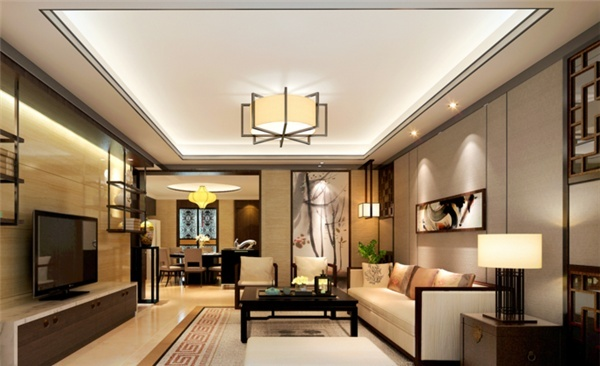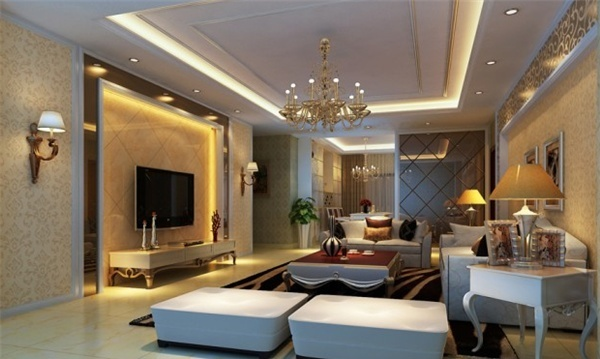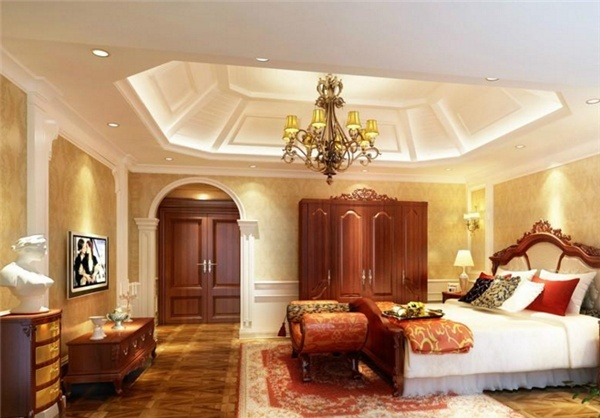|

# 家装灯具的十大品牌排名

在家庭装修的时候，大家往往会结合灯具的装饰性以及灯光的搭配性，装修的效果就是各种元素之间的搭配，由此可以看出大家选购灯具的时候更多的看重的是灯具的装饰性而不是功能性，那么大家知道灯具有哪些品牌呢？下面跟着我们来看看灯具的十大品牌吧。1、华艺

集团拥有20万平方米的灯饰照明产业园，逾两万平方米的总店营业展厅，下辖八家分公司和十四家分厂；雄厚的研发投入确保了华艺灯饰在保持豪华尊贵格调的基础上不断推陈出新，二十年经营累计推出了三万余款尊贵灯饰，成功购建了涵盖灯具、光源、配件等相关产品的研发、生产和销售的系统产业链；精准的市场定位使得华艺灯饰屡屡中标国内外五星级酒店、高档楼盘及政府办公大楼照明工程，进入新世纪以来，集团年销售额再攀新高。

2、奥朵

AOZZO（奥朵），德国IF设计理念在时尚家居领域的灵感臻品，其精髓来自现代设计发源地－德国包毫斯。如今，AOZZO在中国的推广历程已经走过了6年的时间，为无数追求生活品质的中国消费者带来了全新的家居装饰艺术品，并成为卓越品质、摩登设计的代名词。

3、琪朗

中山市琪朗灯饰厂有限公司（简称“琪朗灯饰”）前身为中山市琪朗灯饰厂，创立于1993年，是国内最具实力的现代灯饰企业之一。公司总部位于中山西区沙朗，占地面积20万平方米，注册资本5000万元。琪朗灯饰专注于现代、水晶、欧式等各类民用灯具的研发、生产与销售，产品远销全球100多个国家和地区，在北京、上海等国内120多个大、中城市设立了200多家专卖店。

4、澳克士

中山市澳克士照明电器有限公司位于中国灯饰之都——中山市古镇镇，是古镇最大的灯饰生产厂家——华艺集团的核心企业之一。经过十多年的发展，已发展成为拥有员工1500多名，占地2万多平方米，年产量达三千二百多万支生产能力的灯饰专业厂家。主要生产LED商业照明灯，电子节能灯、吸顶灯、日光灯、支架、电工开关等系列节能环保照明产品。

5、阳光

浙江阳光集团股份有限公司创建于1975年，前身系上虞灯泡厂，1996年改为股份有限公司，2000年7月，“浙江阳光”A股在上海证券交易所挂牌上市，成为国内照明行业首家民营高科技上市企业。浙江阳光集团股份有限公司是国家级重点高新技术企业、国家大型企业、中国主要节能灯生产出口基地之一，同时浙江阳光集团已被列为国家300家重点企业及浙江省重点扶持的大企业集团，国家级重合同、守信用单位，唯一集中国名牌、中国驰名商标、中国出口名牌、中国出口免验于一身的企业。6、佛山照明

佛山电器照明股份有限公司是由佛山市电器照明公司、南海市务庄彩釉砖厂、佛山市鄱阳印刷实业公司共同发起，经广东省企业股份制试点联审小组、广东省经济体制改革委员会批准，通过定向募集方式设立的由法人与自然人混合持股的股份有限公司，成立于1992年10月20日。1993年10月，经中国证监会批准，公司公开发行社会公众股（A 股）1，930万股，并于1993年11月23日在深圳证券交易所挂牌交易。1995年7月23日，公司获准发行5，000万股B 股，并获准上市交易。

7、三雄极光

三雄·极光创立于1991年，一直致力于研发、生产、推广高品质的绿色节能照明产品，为客户提供全方位的照明解决方案和专业服务，是中国最具综合竞争实力的照明品牌之一。公司总部—广东三雄极光照明股份有限公司位于广州，另外在广州、肇庆、重庆等地拥有5大生产基地，年生产LED、荧光灯等照明产品上亿套，涉及商业照明、办公照明、工业照明、户外照明、家居照明等领域。

8、新特丽

新特丽·至惠品牌专卖店是新特丽面向中国广大中等收入人群开发的灯饰产品专门店，除在省会级城市进行拓展外，重点将针对广大二三线城市布点，将更多优质灯饰产品带给更多中国家庭。

9、东方

东方灯饰有限公司始创于1984年08月，座落在“中国灯饰之都”——广东省中山市古镇镇，现工厂占地面积5万多平方米。在苏氏三兄弟的团结协作下，历经30年的艰苦创业，东方灯饰有限公司已具备规模化的生产经营能力，技术力量雄厚；在管理层中，高级工程师、工程师近100名。经过全体员工的不懈努力与开拓创新，东方灯饰在保持同行业领先水平的基础上，已逐步形成“立足灯都、扬名全国、深入海外”的战略格局。

10、开元

中山市开元灯饰有限公司座落于中国经济发达的珠江三角洲地区，被誉为“中国灯饰之都”的广东省中山市古镇镇。 公司始创于2000年1月，经过全体开元人六年多的努力拼搏，已发展成为拥有60000平方米生产厂房及10000平方米销售展厅，员工1500多名的有限责任公司，正在建设并将于年底投入使用的新厂房面积55000平方 米。`声明：本文由入驻焦点开放平台的作者撰写，除焦点官方账号外，观点仅代表作者本人，不代表焦点立场错误信息举报电话： 400-099-0099，邮箱：jubao@vip.sohu.com，或点此进行意见反馈，或点此进行举报投诉。`A B C D E F G H J K L M N P Q R S T W X Y Z
A - B - C - D - E
• A
• 鞍山
• 安庆
• 安阳
• 安顺
• 安康
• 澳门
• B
• 北京
• 保定
• 包头
• 巴彦淖尔
• 本溪
• 蚌埠
• 亳州
• 滨州
• 北海
• 百色
• 巴中
• 毕节
• 保山
• 宝鸡
• 白银
• 巴州
• C
• 承德
• 沧州
• 长治
• 赤峰
• 朝阳
• 长春
• 常州
• 滁州
• 池州
• 长沙
• 常德
• 郴州
• 潮州
• 崇左
• 重庆
• 成都
• 楚雄
• 昌都
• 慈溪
• 常熟
• D
• 大同
• 大连
• 丹东
• 大庆
• 东营
• 德州
• 东莞
• 德阳
• 达州
• 大理
• 德宏
• 定西
• 儋州
• 东平
• E
• 鄂尔多斯
• 鄂州
• 恩施
F - G - H - I - J
• F
• 抚顺
• 阜新
• 阜阳
• 福州
• 抚州
• 佛山
• 防城港
• G
• 赣州
• 广州
• 桂林
• 贵港
• 广元
• 广安
• 贵阳
• 固原
• H
• 邯郸
• 衡水
• 呼和浩特
• 呼伦贝尔
• 葫芦岛
• 哈尔滨
• 黑河
• 淮安
• 杭州
• 湖州
• 合肥
• 淮南
• 淮北
• 黄山
• 菏泽
• 鹤壁
• 黄石
• 黄冈
• 衡阳
• 怀化
• 惠州
• 河源
• 贺州
• 河池
• 海口
• 红河
• 汉中
• 海东
• I
• J
• 晋中
• 锦州
• 吉林
• 鸡西
• 佳木斯
• 嘉兴
• 金华
• 景德镇
• 九江
• 吉安
• 济南
• 济宁
• 焦作
• 荆门
• 荆州
• 江门
• 揭阳
• 金昌
• 酒泉
• 嘉峪关
K - L - M - N - P
• K
• 开封
• 昆明
• 昆山
• L
• 廊坊
• 临汾
• 辽阳
• 连云港
• 丽水
• 六安
• 龙岩
• 莱芜
• 临沂
• 聊城
• 洛阳
• 漯河
• 娄底
• 柳州
• 来宾
• 泸州
• 乐山
• 六盘水
• 丽江
• 临沧
• 拉萨
• 林芝
• 兰州
• 陇南
• M
• 牡丹江
• 马鞍山
• 茂名
• 梅州
• 绵阳
• 眉山
• N
• 南京
• 南通
• 宁波
• 南平
• 宁德
• 南昌
• 南阳
• 南宁
• 内江
• 南充
• P
• 盘锦
• 莆田
• 平顶山
• 濮阳
• 攀枝花
• 普洱
• 平凉
Q - R - S - T - W
• Q
• 秦皇岛
• 齐齐哈尔
• 衢州
• 泉州
• 青岛
• 清远
• 钦州
• 黔南
• 曲靖
• 庆阳
• R
• 日照
• 日喀则
• S
• 石家庄
• 沈阳
• 双鸭山
• 绥化
• 上海
• 苏州
• 宿迁
• 绍兴
• 宿州
• 三明
• 上饶
• 三门峡
• 商丘
• 十堰
• 随州
• 邵阳
• 韶关
• 深圳
• 汕头
• 汕尾
• 三亚
• 三沙
• 遂宁
• 山南
• 商洛
• 石嘴山
• T
• 天津
• 唐山
• 太原
• 通辽
• 铁岭
• 泰州
• 台州
• 铜陵
• 泰安
• 铜仁
• 铜川
• 天水
• 天门
• W
• 乌海
• 乌兰察布
• 无锡
• 温州
• 芜湖
• 潍坊
• 威海
• 武汉
• 梧州
• 渭南
• 武威
• 吴忠
• 乌鲁木齐
X - Y - Z
• X
• 邢台
• 徐州
• 宣城
• 厦门
• 新乡
• 许昌
• 信阳
• 襄阳
• 孝感
• 咸宁
• 湘潭
• 湘西
• 西双版纳
• 西安
• 咸阳
• 西宁
• 仙桃
• 西昌
• Y
• 运城
• 营口
• 盐城
• 扬州
• 鹰潭
• 宜春
• 烟台
• 宜昌
• 岳阳
• 益阳
• 永州
• 阳江
• 云浮
• 玉林
• 宜宾
• 雅安
• 玉溪
• 延安
• 榆林
• 银川
• Z
• 张家口
• 镇江
• 舟山
• 漳州
• 淄博
• 枣庄
• 郑州
• 周口
• 驻马店
• 株洲
• 张家界
• 珠海
• 湛江
• 肇庆
• 中山
• 自贡
• 资阳
• 遵义
• 昭通
• 张掖
• 中卫

1室1厅1厨1卫1阳台

1
2
3
4
5

0
1
2

1

1

0
1
2
3报名成功，资料已提交审核A B C D E F G H J K L M N P Q R S T W X Y Z
A - B - C - D - E
• A
• 鞍山
• 安庆
• 安阳
• 安顺
• 安康
• 澳门
• B
• 北京
• 保定
• 包头
• 巴彦淖尔
• 本溪
• 蚌埠
• 亳州
• 滨州
• 北海
• 百色
• 巴中
• 毕节
• 保山
• 宝鸡
• 白银
• 巴州
• C
• 承德
• 沧州
• 长治
• 赤峰
• 朝阳
• 长春
• 常州
• 滁州
• 池州
• 长沙
• 常德
• 郴州
• 潮州
• 崇左
• 重庆
• 成都
• 楚雄
• 昌都
• 慈溪
• 常熟
• D
• 大同
• 大连
• 丹东
• 大庆
• 东营
• 德州
• 东莞
• 德阳
• 达州
• 大理
• 德宏
• 定西
• 儋州
• 东平
• E
• 鄂尔多斯
• 鄂州
• 恩施
F - G - H - I - J
• F
• 抚顺
• 阜新
• 阜阳
• 福州
• 抚州
• 佛山
• 防城港
• G
• 赣州
• 广州
• 桂林
• 贵港
• 广元
• 广安
• 贵阳
• 固原
• H
• 邯郸
• 衡水
• 呼和浩特
• 呼伦贝尔
• 葫芦岛
• 哈尔滨
• 黑河
• 淮安
• 杭州
• 湖州
• 合肥
• 淮南
• 淮北
• 黄山
• 菏泽
• 鹤壁
• 黄石
• 黄冈
• 衡阳
• 怀化
• 惠州
• 河源
• 贺州
• 河池
• 海口
• 红河
• 汉中
• 海东
• I
• J
• 晋中
• 锦州
• 吉林
• 鸡西
• 佳木斯
• 嘉兴
• 金华
• 景德镇
• 九江
• 吉安
• 济南
• 济宁
• 焦作
• 荆门
• 荆州
• 江门
• 揭阳
• 金昌
• 酒泉
• 嘉峪关
K - L - M - N - P
• K
• 开封
• 昆明
• 昆山
• L
• 廊坊
• 临汾
• 辽阳
• 连云港
• 丽水
• 六安
• 龙岩
• 莱芜
• 临沂
• 聊城
• 洛阳
• 漯河
• 娄底
• 柳州
• 来宾
• 泸州
• 乐山
• 六盘水
• 丽江
• 临沧
• 拉萨
• 林芝
• 兰州
• 陇南
• M
• 牡丹江
• 马鞍山
• 茂名
• 梅州
• 绵阳
• 眉山
• N
• 南京
• 南通
• 宁波
• 南平
• 宁德
• 南昌
• 南阳
• 南宁
• 内江
• 南充
• P
• 盘锦
• 莆田
• 平顶山
• 濮阳
• 攀枝花
• 普洱
• 平凉
Q - R - S - T - W
• Q
• 秦皇岛
• 齐齐哈尔
• 衢州
• 泉州
• 青岛
• 清远
• 钦州
• 黔南
• 曲靖
• 庆阳
• R
• 日照
• 日喀则
• S
• 石家庄
• 沈阳
• 双鸭山
• 绥化
• 上海
• 苏州
• 宿迁
• 绍兴
• 宿州
• 三明
• 上饶
• 三门峡
• 商丘
• 十堰
• 随州
• 邵阳
• 韶关
• 深圳
• 汕头
• 汕尾
• 三亚
• 三沙
• 遂宁
• 山南
• 商洛
• 石嘴山
• T
• 天津
• 唐山
• 太原
• 通辽
• 铁岭
• 泰州
• 台州
• 铜陵
• 泰安
• 铜仁
• 铜川
• 天水
• 天门
• W
• 乌海
• 乌兰察布
• 无锡
• 温州
• 芜湖
• 潍坊
• 威海
• 武汉
• 梧州
• 渭南
• 武威
• 吴忠
• 乌鲁木齐
X - Y - Z
• X
• 邢台
• 徐州
• 宣城
• 厦门
• 新乡
• 许昌
• 信阳
• 襄阳
• 孝感
• 咸宁
• 湘潭
• 湘西
• 西双版纳
• 西安
• 咸阳
• 西宁
• 仙桃
• 西昌
• Y
• 运城
• 营口
• 盐城
• 扬州
• 鹰潭
• 宜春
• 烟台
• 宜昌
• 岳阳
• 益阳
• 永州
• 阳江
• 云浮
• 玉林
• 宜宾
• 雅安
• 玉溪
• 延安
• 榆林
• 银川
• Z
• 张家口
• 镇江
• 舟山
• 漳州
• 淄博
• 枣庄
• 郑州
• 周口
• 驻马店
• 株洲
• 张家界
• 珠海
• 湛江
• 肇庆
• 中山
• 自贡
• 资阳
• 遵义
• 昭通
• 张掖
• 中卫• 手机• 分享
• 设计
免费设计
• 计算器
装修计算器
• 入驻
合作入驻
• 联系
联系我们
• 置顶
返回顶部